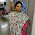## Thursday, May 9, 2013

### ISRO PAPER SOLUTION KEY -2006

Answer key for the ISRO Paper 2006 (SET B) :

1. C

2. C

3. A

4. B

5. D

6. D

7.D

8. A

9. B

10.A

11.A

12.B

13.C

14.C

15.C

16.C

17.D

18.D

19.A

20.B

21.A

22.B

23.A

24.C

25.A

26.B

27.C

28.D

29.D

30.D

31.D

32.A

33.B

34.C

35.B

36.A

37.A

38.D

39.B

40.D

41.D

42.D

43.C

44.

45.C

46.B

47.A

48.

49.D

50.B

51.B

52.A

53.A

54.

55.B

56.

57.B

58.

59.C

60.C

61.D

62.C

63.D

64.D

65.D

66.A

67.B

68.D

69.B

70.C

71.C

72.C

73.A

74.D

75.A

76.B

77.A

78.C

79.D

80.A

If you guys have any doubt . Plz place your comment .We will try to resolve it.

Also If you like the post. Please follow us by joining as member.

1.ans of 30- D .

1.Yes mine answer is also D

2.for 80) option c is also correct.becoz Q=(1/R)*(L/C)^0.5

3.This comment has been removed by the author.

4.can u tell me how to do the 77th problem pls in 2011 isro paper EC setA.

1.suppu ...

Please paste the same question under 2011 solution so that others will also understand...

5.ans 10 --(a)

1.Mine answer is also A. let me know the doubt here

6.Que no 78, ISRO 2006, I am getting answer as 2A, but in the solution is as 4A. I think there is a mistake in redrawing the circuit. Can you please help?

1.Sorry. I got the right answer

7.Que no 76- ISRO 2006, TO FIND THE GAIN OF THE NETWORK
I used the formula, 1+Rf/R1 and Rf=100K and R1=1k. My answer would be approximately 40dB. But here it is given as 20dB. Please tell me where I made the mistake

1.hello priya g,if gain is 100 then it would be 20db by formula 10log(gain)db its 20log(gain) for current or voltage.

8.in q. no. 15 the quantization noise reduces by increasing the sampling frequency but noise density remains the same hence ans ia option A.

9.in q.no. 17 xor gate can be implemented by using 4 two input NAND gates .option C is the correct ans.

10.in q.no. 25 that no. is 3753 in octal format and it can be represented in binary with 9 no.of 1s

11.in q no. 27 ans should be B bcoz flipflop will half the input frequency of 1 hz and duration would be 2 seconds.

12.what was the cutoff in 2006

13.